## Example Questions

### Example Question #1 : How To Find The Length Of A Diagonal Of A Polygon

Polygon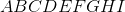is a regular nine-sided polygon, or nonagon, with perimeter 500. Which choice comes closest to the length of diagonal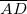?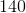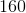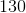Explanation:

Congruent sides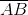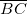, and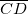, and the diagonalform an isosceles trapezoid.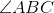and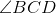. being angles of a nine-sided regular polygon, have measure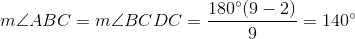The other two angles are supplementary to these: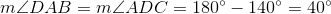The length of one side of the nonagon is one-ninth of 500, so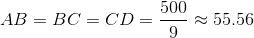The trapezoid formed is below (figure NOT drawn to scale):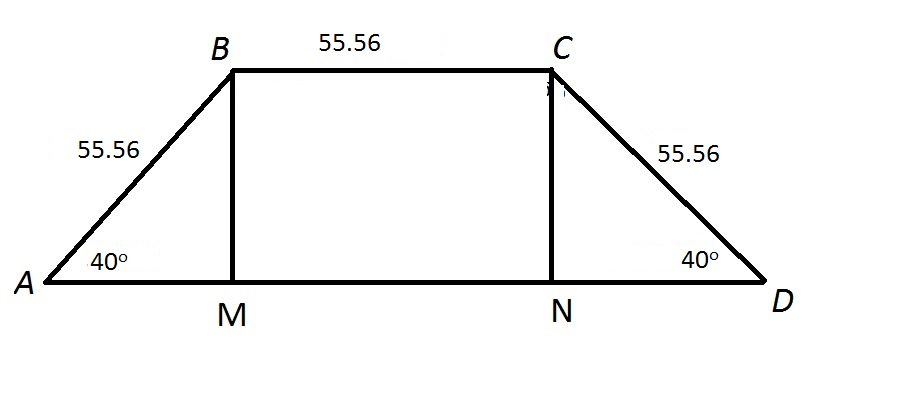Altitudes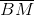and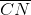to the base have been drawn, so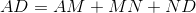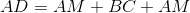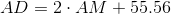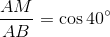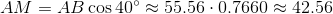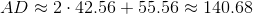This makes 140 the best choice.

### Example Question #2 : How To Find The Length Of A Diagonal Of A Polygon

Polygon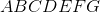is a regular seven-sided polygon, or heptagon, with perimeter 500. Which choice comes closest to the length of diagonal?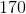Explanation:

Congruent sides, and, and the diagonalform an isosceles trapezoid.and. being angles of a seven-sided regular polygon, have measure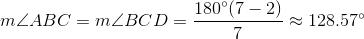The other two angles are supplementary to these: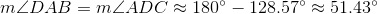The length of one side is one-seventh of 500, so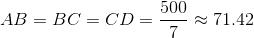The trapezoid formed is below (figure NOT drawn to scale):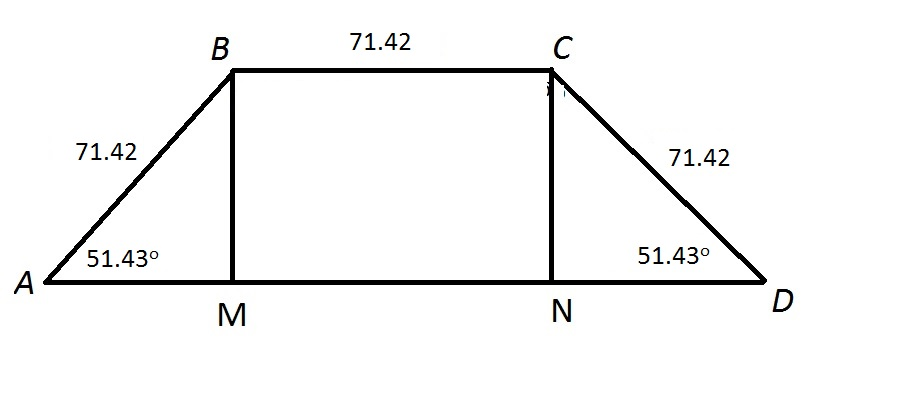Altitudesandto the base have been drawn, so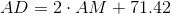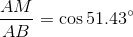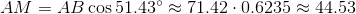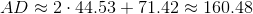This makes 160 the best choice.